# nLab quantum logic gate

Contents

### Context

#### Monoidal categories

monoidal categories

With braiding

With duals for objects

With duals for morphisms

With traces

Closed structure

Special sorts of products

Semisimplicity

Morphisms

Internal monoids

Examples

Theorems

In higher category theory

# Contents

## Idea

Analogously to how a classical logic gate is a function between (finite) sets of tuples of bits (truth values), so a quantum logic gate is a (unitary) linear operator on (finite-dimensional) Hilbert spaces of tensor products of qbits: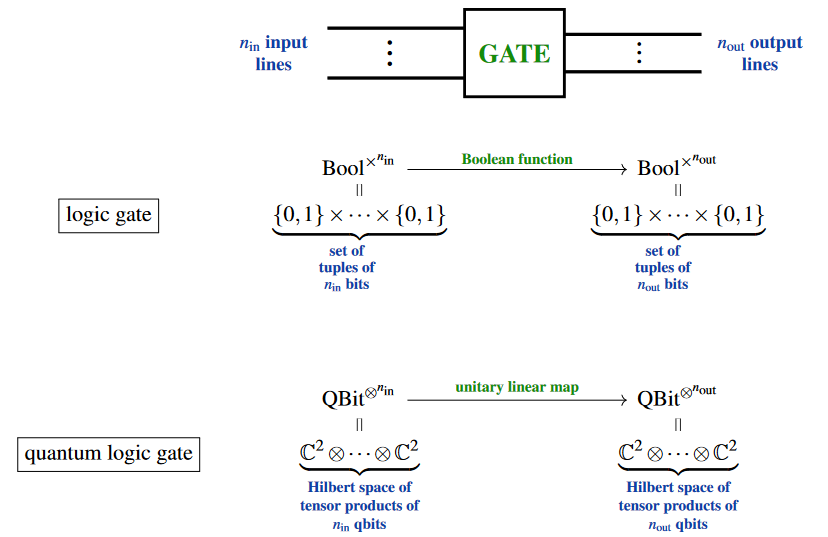Specifically, one calls such a linear map a quantum gate if it is thought of as potentially implemented as a basic operation performed by a quantum computing machine.

As such, typical quantum logic gates operate on a small number of qbits, with more complicated such linear maps obtained by composing a given set of quantum logic gates into quantum logic circuits. Such compilation is hence one model of quantum computation.

## Examples

The first examples are linearizations of classical logic gates, or rather of their reversible versions:

NOT or X: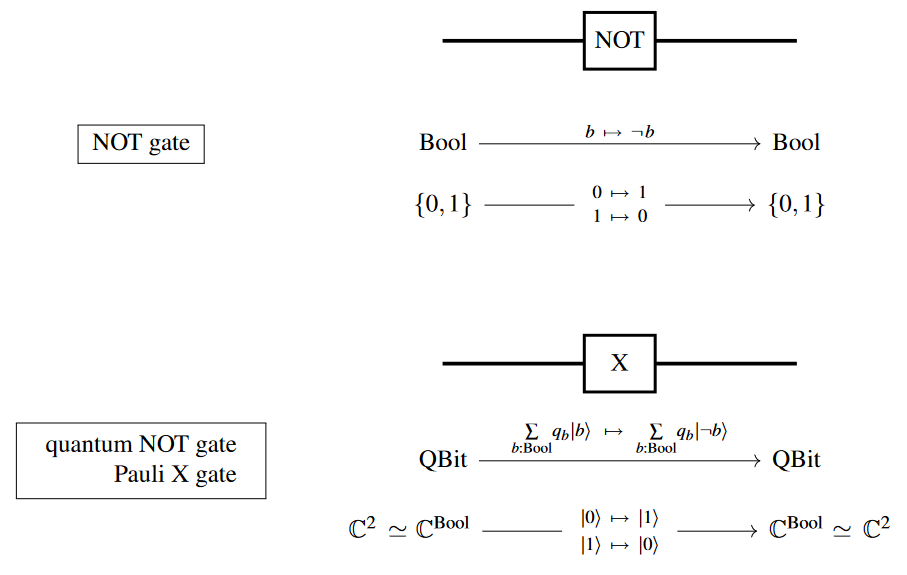XOR and CNOT: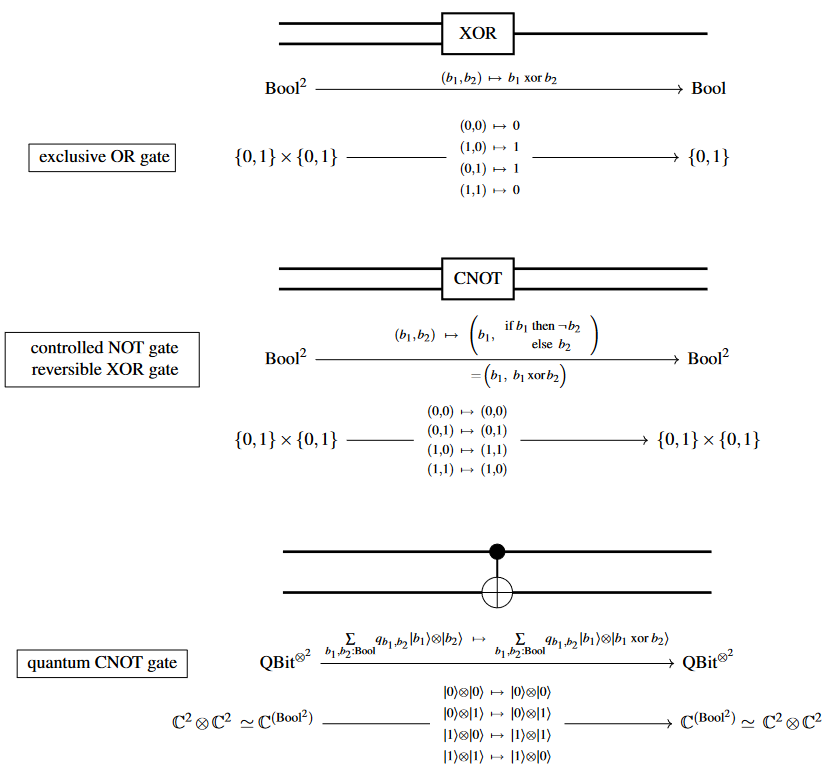AND: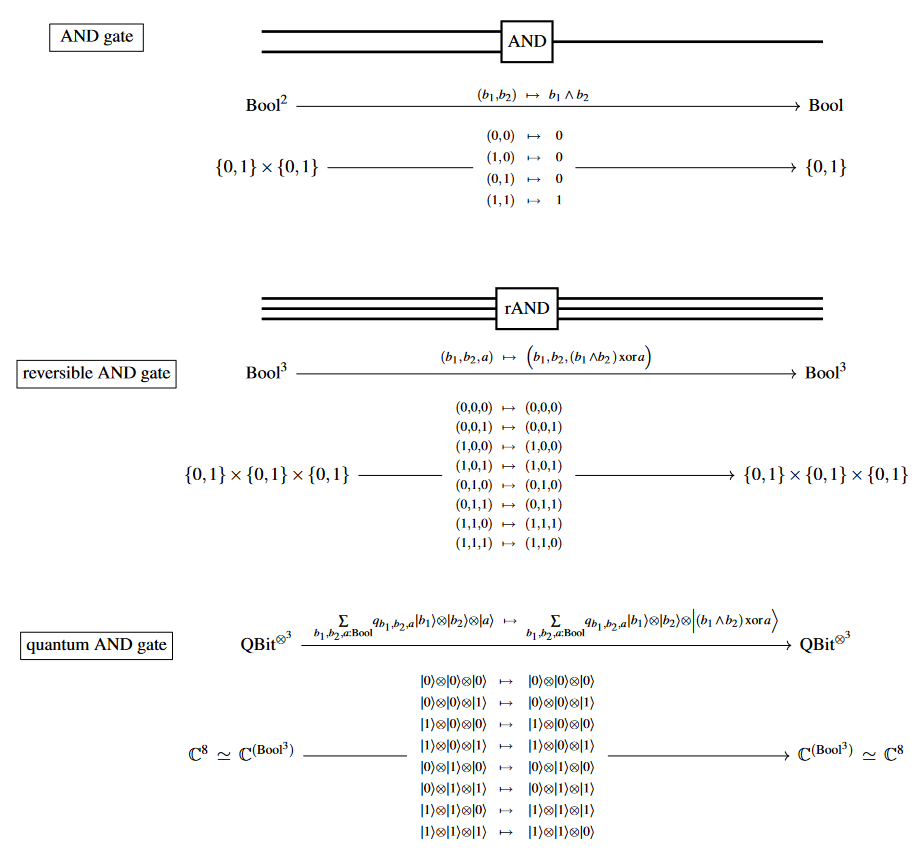The following examples have no classical analog: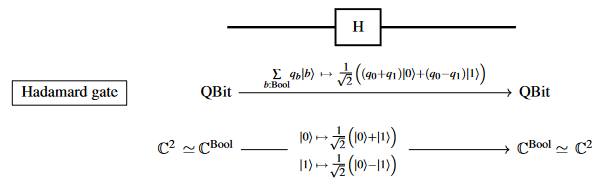Pauli gate

(…)

(…)

The notion of quantum logic gates and quantum circuits originates with